# 2nd PUC Chemistry Question Bank Chapter 4 Chemical Kinetics

You can Download Chapter 4 Chemical Kinetics Questions and Answers, Notes, 2nd PUC Chemistry Question Bank with Answers Karnataka State Board Solutions help you to revise complete Syllabus and score more marks in your examinations.

## Karnataka 2nd PUC Chemistry Question Bank Chapter 4 Chemical Kinetics

### 2nd PUC Chemistry Chemical Kinetics NCERT Textbook Questions and Answers

Question 1.
From the rate expression for the following reactions, determine their order of reaction and the dimensions of the rate constants.
(i) 3NO(g) → N2O (g) Rate = K[NO]2
(ii) H2O2 (aq) + 3I (aq) + 2H+ → 2H2O(l) +I3
Rate = K[H2O2][I]
(iii) CH3CHO (g) → CH4 (g) + CO(g)
Rate = K [CH3CHO]3/2
(iv) C2H5Cl (g) → C2H4 (g) + HCl (g)
Rate = K [C2H5Cl]
Ans:
(i) 2
(ii) 2
(iii) 3/2
(iv) 1

Question 2.
For the reaction:
2A + B → A2B
the rate = k[A][B]2 with k = 2.0 × 10-6 mol-2 L2 s-1. Calculate the initial rate of the reaction when [A] = 0.1 mol L-1, [B] = 0.2 mol L-1. Calculate the rate of reaction after [A] is reduced to 0.06 mol L-1.
Answer:
Initial rate = K [A] [B]2
= 2.0 × 10-6 (mol-2 L2 s-1 × 0.1 (mol-1) × (0.2)2mol2L-2
= 8 × 10-9 mol L-1 s-1
we know that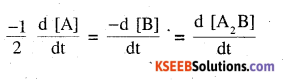$$\frac { 1 }{ 2 }$$ [rate of disappearance of A] = rate of disappearance of B
∴ When [A] is reduced by 0.1 – 0.06 = 0.04 M
[B] is reduced by $$\frac { 1 }{ 2 }$$ × 0.04 = 0.02 M
∴ [B] remaining = 0.2 -0.02 = 0.18 M
Rate of reaction = K [A] [B]2
= 2.0 × 10-6 mol -2 L21
s -1 × 0.06 × (0.18)2 mol3 L-3
= 3.89 × 10-9 Ms-1

Question 3.
The decomposition of NH3on platinum surface is zero order reaction. What are the rates of production of N2 and H2 if k = 2.5 × 10-4(H mol-1L s-1 ?
Answer:
For zero order reaction rate of reaction = K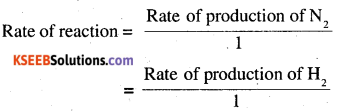∴Rate of production of N2= k = 2.5 × 10-4 Ms-1
Rate of production of H2 = 3k
= 3 × 2.5 × 10-4
= 7.5 × 10-4 Ms-1

Question 4.
The decomposition of dimethyl ether leads to the formation of CH4, H2 and CO and the reaction rate is given by
Rate = K [CH3OCH3]3/2
The rate of reaction is followed by increase in pressure in a closed vessel, so the rate can also be expressed in terms of the partial pressure of dimethyl ether, i.e.
Rate = k (PCH3OCH3)3/2
If the pressure is measured in bar and time in minutes, then what are the units of rate and rate constants?
Answer:
Units rate of reaction = bar min-1
units of rate constant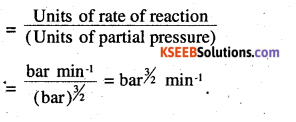Question 5.
Mention the factors that affect the rate of a chemical reaction.
Answer:
(i) Concentration of reactants
(ii) Temperature
(iii) Nature of reactants and products
(iv) Exposure to light (Radiation)
(v) presence of catalysts.

Question 6.
A reaction is second order with respect to a reactant. How is the rate of reaction ‘ affected if the concentration of the reactant is (i) doubled (ii) reduced to half ?
Answer:
(i) Four times
[-rate1 = K[CA]2
CA2 = 2CA
rate2 = K [2CA]2 = 4K [CA]2] = 4 rate1]

(ii) 1/4 times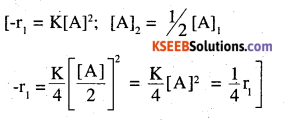Question 7.
What is the effect of temperature on the rate constant of a reaction ? How can this temperature effect on rate constant be represented quantitatively.
Answer:
Increasing the temperature on decreasing the activation energy will result in an increase in the rate of reaction and an exponential increase in the rate constant. On increasing the temperature the fraction of molecules which collide with energy greater than Ea increases and hence the rate constant (exponentially)
K = A -ea/RT , quantitative representation of temperature effect on rate constant.

Question 8.
In a pseudo first order hydrolysis of ester in water, the following results were obtained:

 t/s 0 30 60 90 [Ester]/mol L-1 0.55 0.31 0.17 0.085

(i) Calculate the average rate of reaction between the time interval 30 to 60 seconds.
(ii) Calculate the pseudo first order rate constant for the hydrolysis of ester.
Answer:
(i)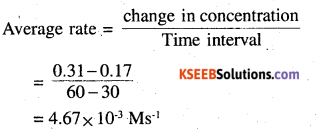(ii) For pseudo first order reaction,
Average rate = Rate constant × Average concentration Average rate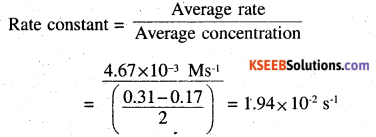Question 9.
A reaction is first order in A and second order in B.
(i) Write the differential rate equation.
(ii) How is the rate affected on increasing the concentration of B three times?
(iii) How is the rate affected when the concentrations of both A and B are doubled?
Answer:
(i)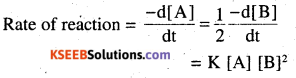(ii) When concentration of B is tripled, rate of reaction increases by 9 times.
(iii) Rate of reaction increases by 8 times.

Question 10.
In a reaction between A and B, the initial rate reaction (r0) was measured for different initial concentrations of A and B as given below.

 A/ mol L-1 0.20 0.20 0.40 B/ mol L-1 0.30 0.10 0.05 rθmol L-1S-5 5.07×10-5 5.07×10-5 1.43 x 10-5

what is the order of the reaction with respect to A and B ?
Answer:
Let the rate law be, r = K [A]x [B]y
r1 = K[0.2]x[0.3]y = 5.07 × 10-5 -(1) .
r2 = K[0.2]x [0.1]y = 5.07 ×10-5 -(2)
r3 = K [0.4]x [0.05]y = 7.6 × 10-5 -(3) ,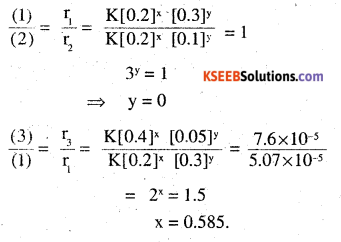∴ The order of reaction with respect to A is 0.585 and with respect to B is 0.
∴ rate law = K[A]0.858 [B]0 = K [A]0.585.

Question 11.
The following results have been obtained during the kinetic studies of the reaction 2A + B → C + D

 Experiment [A]/mol L-1 [B]/mol L-1 Initial rate of formation of D/mol L-1min-1 I 0.1 0.1 6.0 x 10-3 II 0.3 0.2 7.2 x 10-2 III 0.3 0.4 2.88 x 10-1 IV 0.4 0.1 2.40 x 10-2

Determine the rate law and the rate constant for the reaction.
Answer:
r = K [A]x [B]y
r, = K[0.1]x [0.1]y = 6.0 × 10-3 -(i)
r2 = K [0.3]x [0.2]y = 7.2 × 10-2 -(2)
r3 = K [0.3]x [0.4]y = 2.88 × 10-1 -(3)
r4 = K [0.4]x [Q.l]y = 2.4 × 10-2 -(4)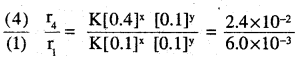4x = 4
⇒ x =12y = 4 ⇒ y = 2.

∴ Rate law, r = K [A]1 [B]2
Rate constant,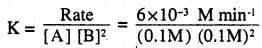= 6M-2min-1Question 12.
The reaction between A and B is first order with respect to A and zero order with respect to B. Fill in the blanks in the following table:

 Experiment [A]/mol L-1 [B]/mol L-1 Initial rate /mol L-1 min-1 I 0.1 0.1 2.0 x 10-2 II x 0.2 4.0 x 10-2 III 0.4 0.4 y IV z 0.2 2.0 x 10-2

Answer:
rate law = K [A]
from I 2.0 × 10-2 = K 0.1
⇒ K = 2.0 × 10-1min-1
from II 4 .0 × 10-2 = 2 .0 × 10-31 x
⇒ K = 2 .0 × 10-1 mol L-1
from III y = 2.0 × 10-1 × 0.4
⇒ y = 8 × 10-2 mol 1L
from IV 2.0 × 10-2 = 2.0 × 10-1 × z
⇒ z = 0.1 mol L-1.

Question 13.
Calculate the half – life of a first order reaction from their rate constants given below.
(i) 200 s-1
(ii) 2 min-1
(iii) 4 years-1
Answer: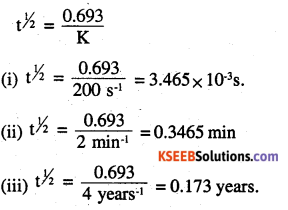Question 14.
The half-life for radioactive decay of 14C is 5730 years. An archaeological artifact containing wood had only 80% of the 14C found in a living tree. Estimate the age of the sample.
Answer:
[A]0 = 100
[A] = 80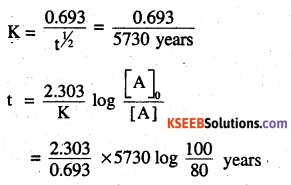= 1845 years
∴The age of the sample is 1845 years.

Question 15.
The rate constant for a first order reaction is 60 s-1. How much time will it take to reduce the initial concentration of the reactant to its 1/16 th value?
Answer:
K = 60 s-1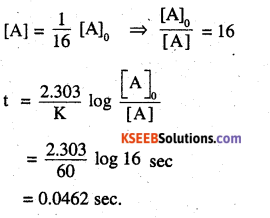Question 16.
During nuclear explosion, one of the products is MSr with half-life of 28.1 years. If mg of 90Sr was absorbed in the bones of a newly born baby instead of calcium, how much of it will remain after 10 years and 60 years if it is not lost metabolically ?
Answer:
t1/2 = 28.1 years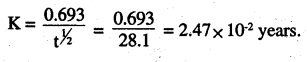[A]0 = 2.47 × 1μg
For 10 years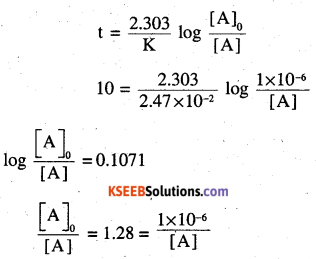[A] =0.78 × 10-6g = 0.78μg
For 60 years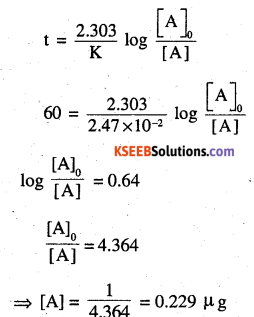The amount of 90Sr that will remain in the child’s body after 10 years is 0.78μg and after 60 years is 0.229 μg.

Question 17.
For a first order reaction, show that time required for 99% completion is twice the time required for the completion of 90% of reaction.
Answer: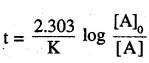for 99% completion,
if [A]0 = 100
[A] = 100-99= 1
for 90% completion
[A] = 100-99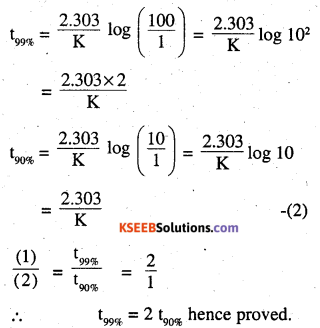Question 18.
A first order reaction takes 40 min for 30% decomposition. Calculate t1/2.
Answer:
[A]0 = 100.
[A] = 100 – 30 = 70
t = 40min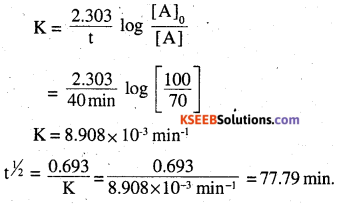Question 19.
The rate constant for the decomposition of hydrocarbons is 2.418 × 10-5s-1 at 546 K. If the energy of activation is 179.9 kJ/mol, what will be the value of pre-exponential factor ?
Answer:
Pre – exponential factor = A = Arrhenius
factor = frequency factor
K = 2.418 × 10-5s-1
T = 546 K.
Ea= 179.9 × 10-3J mol-1
[By Arrhenius equation] K = $$\text { Ae }^{-\mathrm{E}} / \mathrm{kr}$$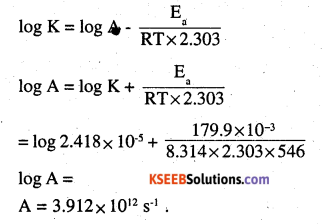Question 20.
onsider a certain reaction A → Products with k = 2.0 × 10-2 s-1. Calculate the concentration of A remaining after 100s if the initial concentration of A is 1.0 mol Lr1. Ans: [A]0 = 1 mol L-1
Answer:
[A]0 = 1 mol L-1
t = 100s
k = 2.0 × 10-2s-1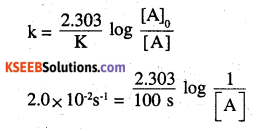log [A] = -0.8684
⇒ [A] = 10 -0.8684 = 0.354 mol L-1Question 21.
Sucrose decomposes in acid solution into glucose and fructose according to the first order rate law, with t1/2 = 3.00 hours. What fraction of sample of sucrose remains after 8 hours ?
Answer:
t1/2 = 3 hrs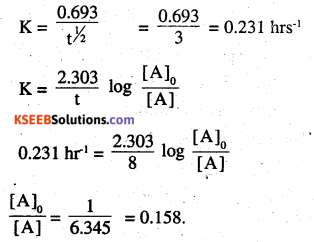Question 22.
The decomposition of hydrocarbon follows the equation K = (1.5 × 1011 s-1)
$$e^{\frac{-28000 K}{T}}$$ Calculate Ea
Answer:
According to Arrehenius equation
K = Ae-Ea/RT
Given K = (4.5 × 1011s-1)$$e^{\frac{-28000 K}{T}}$$
comparing the two equations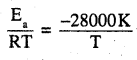Ea = 28000 × R
= 28000 × 8.314 J mol-1
= 232.79 KJ mol-1.

Question 23.
The rate constant for the first order decomposition of H2O2 is given by the following equation:
log k = 14.34 – $$\frac{1.25 \times 10^{4} K}{\mathbf{T}}$$
Calculate Ea for this reaction and at what temperature will Its half-period be 256 minutes?
Answer:
According to Arrehenius equation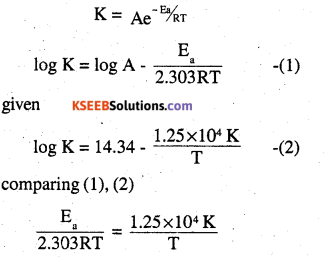Ea = 2.303 R × 1.25 × 104 K
= 2.303 × 8.3l4 JK-1 mol-1 × 1.25 × 104 K
= 239.34 KJ mol-1
What t1/2 = 356 min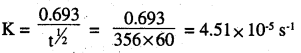Substituting K in given equation
log(4.51 × 10-5) = 14.34 – $$\frac{1.25 \times 10^{4}}{\mathrm{T}}$$
T = 669 K.

Question 24.
The decomposition of A into product has value of k as 4.5 × 103 s-1 at 10°C and energy of activation 60 kj mol-1. At what temperature would k be 1.5 × 104s-1 ?
Answer:
k1 = 4.5 × 103 s-1
k2 = 1.5 × 104s-1
Ea = 60 × 103 J mol-1
R = 8.314 JK-1 mol-1
T1 = 283 k
T2 = ?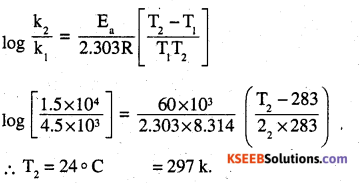Question 25.
The rate of a reaction quadruples when the temperature changes from 293 K to 313 K. Calculate the energy of activation of the reaction assuming that it does not change with temperature.
Answer:
T1 = 283 k
T2 = 313 k
k2 = 4k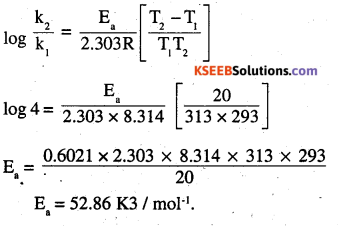### 2nd PUC Chemistry Chemical Kinetics Additional Questions and Answers

Question 1.
Name the factors on which the rate of a particular reaction depends. (H. S .B 2001)
Answer:
Concentration, temperature, presence of catalyst and light, surface area of reactants.

Question 2.
Write the expression for rate of reaction in terms of each reactant and product for the reaction.
N2 + 3H2 → 2NH3 (P.S.B 2011, HSB 2000)
Answer: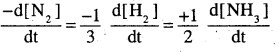Question 3.
Give the example of a reaction having fractional order. (PSB 2000)
Answer:
Decomposition of acetal dehyde (order =1.5)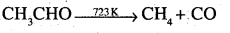Question 4.
Give an example of a psueudo first order reaction. (AISB, DSB 2004, DSB 2006)
Answer:
Acid catalysed hydrolysis of ethyl acetate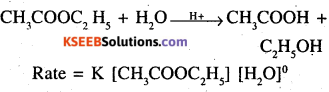Rate = K [CH3COOC2H5] [H20]°

Question 5.
For an elementary reaction,
2 A + B → 3C
the rate of appearance of C at time ‘t’ is 1.3 × 104 mol-1 S-1. Calculate at this time
(i) Rate of the reaction
(ii) Rate of disappearance of A. (CBSE sample paper II 2008)
Answer:
Rate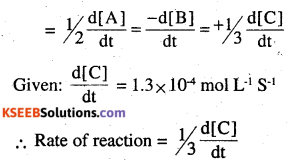= 1/3 × 1.3 × 10-4mol L-1S-1
= 4.33 × 10-5 mol L-1S-1

Rate of disappearance of A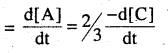2/3 × 1.3 × 10-4 L-1S-1
= 8.66 × 10-5 mol L-1S-1

Question 6.
The rate law for a reaction is found to be
Rate = K [NO2] [I ] [H+]2 How would the rate of reaction change when
(i) Concentration of H+ is doubled
(ii) Concentration of I is halved
(iii) Concentration of each of NO2, I and H+ are tripled ?
Answer:
Suppose initially the concentration are [NO2] = a mol L-1, [I ] = b mol L and [H+] = c mol L-1
∴ Rate = K abc2

(i) New [H+] = 2c
∴ New rate = 4ab (2c)2 = 4abc2
= 4 times

(ii) New [I ] = $$\frac { b }{ 2 }$$
New Rate = Ka$$\frac { b }{ 2 }$$c2= $$\frac { 1 }{ 2 }$$ Kabc2
ie. rate of reaction is halved

(iii) New [NO2] = 3a, [I ] = 3b, [H+] = 3c
New rate = K (3a) (3b) (3c)2
= 81K abc2 = 81 times.

Question 7.
The decomposition of NH3 on platinum surface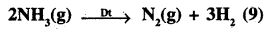is zero order with k = 2.5 × 10-4 mol L-1 S-1
What are the rates of production of N2 and H2
Answer:
2NH3 -> N2 + 3H2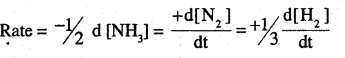For zero order reaction, rate = K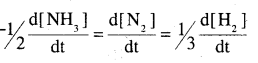= 2.5 × 10-4 mol L-1 S-1
Rate of production of H2 = $$\frac{\mathrm{d}\left[\mathrm{H}_{2}\right]}{\mathrm{dt}}$$
= 3 × (2.5 × 10-4 mol L-1 S-1)
= 7.5 × 10-4 mol -1 S-1Question 8.
Why does coal not burn by itself in air, but once initiated by a flame, continues to bum ?
Answer:
Activation energy for the combustion reaction is very high and is not available at room temperature. Oh applying flame, to a part of the coal and air in contact with the flame absorb heat which provides the necessary activation energy and combustion starts. The heat liberated further provides activation energy for the combustion to continue.

Question 9.
Following reaction takes place in one step:
2NO(g) + O2(g) ⇌ 2NO2(g)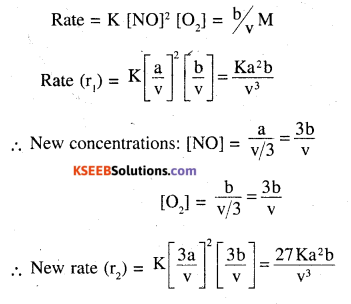$$\frac{\mathbf{r}_{2}}{\mathbf{r}_{1}}$$ = 27 or r2 = 27r1 ie rate becomes 27
times
There is no effect on the order of reaction.

Question 10.
Rate constant K of a reaction varies with temperature according to the equation
log K = constant . $$\frac{\mathbf{E} \mathbf{a}}{2.303 \mathbf{R}} \frac{\mathbf{1}}{\mathbf{T}}$$
Answer:
where Ea is the energy of activation for the reaction. When a graph is plotted for
log K versus$$\frac { 1 }{ T }$$, a straight line with a slope 6670 K is obtained. Calculate the energy of activation for this reaction. State units (R = 8.314JK-1 mol-1)
Answer:
Slope of the lin,e
$$\frac{-E a}{2.303 R}$$ =-6670K
Ea = 2.303 × 8.314 (JK-1 mol-1) × 6670K
= 127711.4J mol-1.